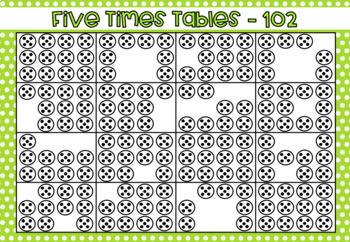# Five Times Tables Spinner Games - Game Based Learning;
3rd - 6th
Subjects
Resource Type
Standards
Formats Included
• PDF
Pages
15 pages
Report this resource to TPT
##### Also included in
1. NumeracyThis BUNDLE includes: - 2 Times Tables (x5 games - leveled) - 3 Times Tales (x5 games - leveled) - 4 Times Tables (x5 games - leveled) - 5 Times Tables (x5 games - leveled) - 6 Times Tables (x5 games - leveled) - 7 Times Tables (x5 games - leveled) - 8 Times Tables (x5 games - leveled) - 9 T
Price \$15.00Original Price \$20.00Save \$5.00

### Description

Numeracy

These SPINNER GAMES have been leveled and are ideal for differentiation in your class to cater for mixed-ability groups, as well as ensuring that learners at different starting points can receive the instruction they need to GROW and SUCCEED!

The first two levels (boards 101 & 102) contain the terms 'groups of' and a pictorial representation of this, which consolidates the understanding of multiplication. Also, if you're currently focusing on subitising, then these two games are for you!

Levels 3 and 4 (boards 103 & 104) contain the symbol used for multiplication, as well as numerals for the answers.

As a BONUS, this resource also includes a game entitled 'Commutative Property Practice.' This game allows students to become familiar with the commutative property where you can multiply numbers in any order and the answer will always be the same.

Australian Curriculum: Numeracy Year 3

Recall multiplication facts of two, three, five and ten and related division facts (ACMNA056)

Head on over to my Instagram account @_miss_carlee_ where I've saved a video of this resource under the Instagram Highlights: 'Math is Fun.'

A QUICK TIP FOR YOU...

Did you know that you can earn TpT credits to use for future purchases? Go to your 'My Purchases' page and next to each product that you've already purchased, you will notice a 'Provide Feedback' button. Click on it and take a minute to provide some positive feedback! Every time you do this, TpT rewards you with credits, which you can use as a deduction toward future purchases!

I highly value your feedback and comments. It not only warms my heart, but it helps me to determine which resources are most valuable for teachers. I always look forward to creating new products and supporting you in the classroom!

Total Pages
15 pages
N/A
Teaching Duration
N/A
Report this resource to TPT
Reported resources will be reviewed by our team. Report this resource to let us know if this resource violates TPT’s content guidelines.

### Standards

to see state-specific standards (only available in the US).
Interpret products of whole numbers, e.g., interpret 5 × 7 as the total number of objects in 5 groups of 7 objects each. For example, describe a context in which a total number of objects can be expressed as 5 × 7.
Apply properties of operations as strategies to multiply and divide. Examples: If 6 × 4 = 24 is known, then 4 × 6 = 24 is also known. (Commutative property of multiplication.) 3 × 5 × 2 can be found by 3 × 5 = 15, then 15 × 2 = 30, or by 5 × 2 = 10, then 3 × 10 = 30. (Associative property of multiplication.) Knowing that 8 × 5 = 40 and 8 × 2 = 16, one can find 8 × 7 as 8 × (5 + 2) = (8 × 5) + (8 × 2) = 40 + 16 = 56. (Distributive property.)
Fluently multiply and divide within 100, using strategies such as the relationship between multiplication and division (e.g., knowing that 8 × 5 = 40, one knows 40 ÷ 5 = 8) or properties of operations. By the end of Grade 3, know from memory all products of two one-digit numbers.
Identify arithmetic patterns (including patterns in the addition table or multiplication table), and explain them using properties of operations. For example, observe that 4 times a number is always even, and explain why 4 times a number can be decomposed into two equal addends.